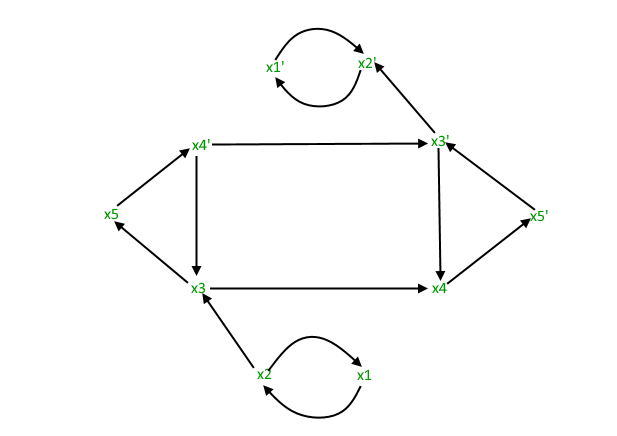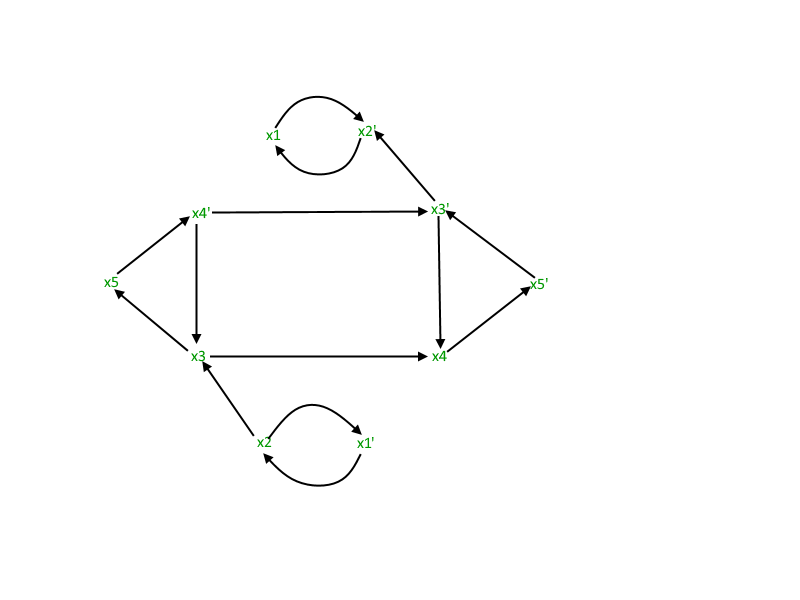# 2-Satisfiability (2-SAT) Problem

• Difficulty Level : Medium
• Last Updated : 14 Jul, 2022

## Boolean Satisfiability Problem

Boolean Satisfiability or simply SAT is the problem of determining if a Boolean formula is satisfiable or unsatisfiable.

• Satisfiable : If the Boolean variables can be assigned values such that the formula turns out to be TRUE, then we say that the formula is satisfiable.
• Unsatisfiable : If it is not possible to assign such values, then we say that the formula is unsatisfiable.

Examples:

•, is satisfiable, because A = TRUE and B = FALSE makes F = TRUE.
•, is unsatisfiable, because:

Note : Boolean satisfiability problem is NP-complete (For proof, refer Cook’s Theorem).## What is 2-SAT Problem

2-SAT is a special case of Boolean Satisfiability Problem and can be solved
in polynomial time.

To understand this better, first let us see what is Conjunctive Normal Form (CNF) or also known as Product of Sums (POS).

CNF : CNF is a conjunction (AND) of clauses, where every clause is a disjunction (OR).
Now, 2-SAT limits the problem of SAT to only those Boolean formula which are expressed as a CNF with every clause having only 2 terms(also called 2-CNF).

Example:Thus, Problem of 2-Satisfiability can be stated as:

Given CNF with each clause having only 2 terms, is it possible to assign such values to the variables so that the CNF is TRUE?

Examples:

Input :Output : The given expression is satisfiable. (for x1 = FALSE, x2 = TRUE)Input :Output : The given expression is unsatisfiable. (for all possible combinations of x1 and x2)

## Approach for 2-SAT Problem

For the CNF value to come TRUE, value of every clause should be TRUE. Let one of the clause be.= TRUE

• If A = 0, B must be 1 i.e.• If B = 0, A must be 1 i.e.Thus,= TRUE is equivalent toNow, we can express the CNF as an Implication. So, we create an Implication Graph which has 2 edges for every clause of the CNF.is expressed in Implication Graph asThus, for a Boolean formula with ‘m’ clauses, we make an Implication Graph with:

• 2 edges for every clause i.e. ‘2m’ edges.
• 1 node for every Boolean variable involved in the Boolean formula.

Let’s see one example of Implication Graph.Note: The implication (if A then B) is equivalent to its contrapositive (ifthen).
Now, consider the following cases:

CASE 1: If[Tex]exists in the graph[/Tex]This meansIf X = TRUE,= TRUE, which is a contradiction.But if X = FALSE, there are no implication constraints.Thus, X = FALSE

CASE 2: If[Tex]exists in the graph[/Tex]This meansIf= TRUE, X = TRUE, which is a contradiction.But if= FALSE, there are no implication constraints.Thus,= FALSE i.e. X = TRUE

CASE 3: If[Tex]both exist in the graph[/Tex]One edge requires X to be TRUE and the other one requires X to be FALSE.Thus, there is no possible assignment in such a case.

CONCLUSION:

If any two variablesandare on a cycle i.e.both exists, then the CNF is unsatisfiable. Otherwise, there is a possible assignment and the CNF is satisfiable.
Note here that, we use path due to the following property of implication:
If we haveThus, if we have a path in the Implication Graph, that is pretty much same as having a direct edge.

CONCLUSION FROM IMPLEMENTATION POINT OF VIEW:

If both X andlie in the same SCC (Strongly Connected Component), the CNF is unsatisfiable.
A Strongly Connected Component of a directed graph has nodes such that every node can be reach from every another node in that SCC.
Now, if X andlie on the same SCC, we will definitely havepresent and hence the conclusion.
Checking of the SCC can be done in O(E+V) using the Kosaraju’s Algorithm

Implementation:

## C++

 // C++ implementation to find if the given// expression is satisfiable using the// Kosaraju's Algorithm#include using namespace std;  const int MAX = 100000;  // data structures used to implement Kosaraju's// Algorithm. Please refer// https://www.geeksforgeeks.org/strongly-connected-components/vector<int> adj[MAX];vector<int> adjInv[MAX];bool visited[MAX];bool visitedInv[MAX];stack<int> s;  // this array will store the SCC that the// particular node belongs toint scc[MAX];  // counter maintains the number of the SCCint counter = 1;  // adds edges to form the original graphvoid addEdges(int a, int b){    adj[a].push_back(b);}  // add edges to form the inverse graphvoid addEdgesInverse(int a, int b){    adjInv[b].push_back(a);}  // for STEP 1 of Kosaraju's Algorithmvoid dfsFirst(int u){    if(visited[u])        return;      visited[u] = 1;      for (int i=0;i b[i]        // AND -b[i] -> a[i]        if (a[i]>0 && b[i]>0)        {            addEdges(a[i]+n, b[i]);            addEdgesInverse(a[i]+n, b[i]);            addEdges(b[i]+n, a[i]);            addEdgesInverse(b[i]+n, a[i]);        }          else if (a[i]>0 && b[i]<0)        {            addEdges(a[i]+n, n-b[i]);            addEdgesInverse(a[i]+n, n-b[i]);            addEdges(-b[i], a[i]);            addEdgesInverse(-b[i], a[i]);        }          else if (a[i]<0 && b[i]>0)        {            addEdges(-a[i], b[i]);            addEdgesInverse(-a[i], b[i]);            addEdges(b[i]+n, n-a[i]);            addEdgesInverse(b[i]+n, n-a[i]);        }          else        {            addEdges(-a[i], n-b[i]);            addEdgesInverse(-a[i], n-b[i]);            addEdges(-b[i], n-a[i]);            addEdgesInverse(-b[i], n-a[i]);        }    }      // STEP 1 of Kosaraju's Algorithm which    // traverses the original graph    for (int i=1;i<=2*n;i++)        if (!visited[i])            dfsFirst(i);      // STEP 2 of Kosaraju's Algorithm which    // traverses the inverse graph. After this,    // array scc[] stores the corresponding value    while (!s.empty())    {        int n = s.top();        s.pop();          if (!visitedInv[n])        {            dfsSecond(n);            counter++;        }    }      for (int i=1;i<=n;i++)    {        // for any 2 variable x and -x lie in        // same SCC        if(scc[i]==scc[i+n])        {            cout << "The given expression "                 "is unsatisfiable." << endl;            return;        }    }      // no such variables x and -x exist which lie    // in same SCC    cout << "The given expression is satisfiable."         << endl;    return;}  //  Driver function to test above functionsint main(){    // n is the number of variables    // 2n is the total number of nodes    // m is the number of clauses    int n = 5, m = 7;      // each clause is of the form a or b    // for m clauses, we have a[m], b[m]    // representing a[i] or b[i]      // Note:    // 1 <= x <= N for an uncomplemented variable x    // -N <= x <= -1 for a complemented variable x    // -x is the complement of a variable x      // The CNF being handled is:    // '+' implies 'OR' and '*' implies 'AND'    // (x1+x2)*(x2’+x3)*(x1’+x2’)*(x3+x4)*(x3’+x5)*    // (x4’+x5’)*(x3’+x4)    int a[] = {1, -2, -1, 3, -3, -4, -3};    int b[] = {2, 3, -2, 4, 5, -5, 4};      // We have considered the same example for which    // Implication Graph was made    is2Satisfiable(n, m, a, b);      return 0;}

## Java

 // Java implementation to find if the given // expression is satisfiable using the // Kosaraju's Algorithm import java.io.*;import java.util.*;  class GFG{  static final int MAX = 100000;  // Data structures used to implement Kosaraju's// Algorithm. Please refer// https://www.geeksforgeeks.org/strongly-connected-components/@SuppressWarnings("unchecked")static List > adj = new ArrayList();  @SuppressWarnings("unchecked")static List > adjInv = new ArrayList();static boolean[] visited = new boolean[MAX];static boolean[] visitedInv = new boolean[MAX];static Stack s = new Stack();  // This array will store the SCC that the// particular node belongs tostatic int[] scc = new int[MAX];  // counter maintains the number of the SCCstatic int counter = 1;  // Adds edges to form the original graph voidstatic void addEdges(int a, int b){    adj.get(a).add(b);}  // Add edges to form the inverse graphstatic void addEdgesInverse(int a, int b){    adjInv.get(b).add(a);}  // For STEP 1 of Kosaraju's Algorithmstatic void dfsFirst(int u){    if (visited[u])        return;      visited[u] = true;      for(int i = 0; i < adj.get(u).size(); i++)        dfsFirst(adj.get(u).get(i));      s.push(u);}  // For STEP 2 of Kosaraju's Algorithmstatic void dfsSecond(int u){    if (visitedInv[u])        return;      visitedInv[u] = true;      for(int i = 0; i < adjInv.get(u).size(); i++)        dfsSecond(adjInv.get(u).get(i));      scc[u] = counter;}  // Function to check 2-Satisfiabilitystatic void is2Satisfiable(int n, int m,                            int a[], int b[]){          // Adding edges to the graph    for(int i = 0; i < m; i++)     {                  // variable x is mapped to x        // variable -x is mapped to n+x = n-(-x)          // for a[i] or b[i], addEdges -a[i] -> b[i]        // AND -b[i] -> a[i]        if (a[i] > 0 && b[i] > 0)         {            addEdges(a[i] + n, b[i]);            addEdgesInverse(a[i] + n, b[i]);            addEdges(b[i] + n, a[i]);            addEdgesInverse(b[i] + n, a[i]);        }          else if (a[i] > 0 && b[i] < 0)         {            addEdges(a[i] + n, n - b[i]);            addEdgesInverse(a[i] + n, n - b[i]);            addEdges(-b[i], a[i]);            addEdgesInverse(-b[i], a[i]);        }          else if (a[i] < 0 && b[i] > 0)         {            addEdges(-a[i], b[i]);            addEdgesInverse(-a[i], b[i]);            addEdges(b[i] + n, n - a[i]);            addEdgesInverse(b[i] + n, n - a[i]);        }          else        {            addEdges(-a[i], n - b[i]);            addEdgesInverse(-a[i], n - b[i]);            addEdges(-b[i], n - a[i]);            addEdgesInverse(-b[i], n - a[i]);        }    }      // STEP 1 of Kosaraju's Algorithm which    // traverses the original graph    for(int i = 1; i <= 2 * n; i++)        if (!visited[i])            dfsFirst(i);      // STEP 2 of Kosaraju's Algorithm which    // traverses the inverse graph. After this,    // array scc[] stores the corresponding value    while (!s.isEmpty())     {        int top = s.peek();        s.pop();          if (!visitedInv[top])         {            dfsSecond(top);            counter++;        }    }      for(int i = 1; i <= n; i++)     {                  // For any 2 variable x and -x lie in        // same SCC        if (scc[i] == scc[i + n])        {            System.out.println("The given expression" +                               "is unsatisfiable.");            return;        }    }      // No such variables x and -x exist which lie    // in same SCC    System.out.println("The given expression " +                        "is satisfiable.");}  // Driver codepublic static void main(String[] args){          // n is the number of variables    // 2n is the total number of nodes    // m is the number of clauses    int n = 5, m = 7;      for(int i = 0; i < MAX; i++)     {        adj.add(new ArrayList());        adjInv.add(new ArrayList());    }      // Each clause is of the form a or b    // for m clauses, we have a[m], b[m]    // representing a[i] or b[i]      // Note:    // 1 <= x <= N for an uncomplemented variable x    // -N <= x <= -1 for a complemented variable x    // -x is the complement of a variable x      // The CNF being handled is:    // '+' implies 'OR' and '*' implies 'AND'    // (x1+x2)*(x2â+x3)*(x1â+x2â)*(x3+x4)*(x3â+x5)*    // (x4â+x5â)*(x3â+x4)    int a[] = { 1, -2, -1, 3, -3, -4, -3 };    int b[] = { 2, 3, -2, 4, 5, -5, 4 };      // We have considered the same example     // for which Implication Graph was made    is2Satisfiable(n, m, a, b);}}  // This code is contributed by jithin

Output

The given expression is satisfiable.

More Test Cases:

Input : n = 2, m = 3
a[] = {1, 2, -1}
b[] = {2, -1, -2}
Output : The given expression is satisfiable.

Input : n = 2, m = 4
a[] = {1, -1, 1, -1}
b[] = {2, 2, -2, -2}
Output : The given expression is unsatisfiable.

This article is contributed by Aanya Jindal. If you like GeeksforGeeks and would like to contribute, you can also write an article using write.geeksforgeeks.org or mail your article to review-team@geeksforgeeks.org. See your article appearing on the GeeksforGeeks main page and help other Geeks.

My Personal Notes arrow_drop_up